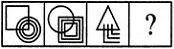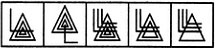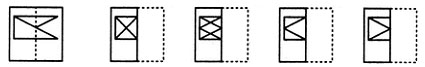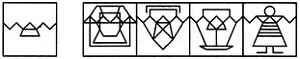# Score Well in the Visual Reasoning Section of MAH CET

The MAH-CET exam has approximately 25 - 30 questions of visual reasoning. Visual reasoning, which has a rare appearance in the other entrance exams at present, forms a significant proportion of this exam. As a total of around 15% questions of the total test are from this area, practicing these questions is very important. There is no negative marking. The difficulty level of questions in this category is from easy to moderate. But considering that you would not have practiced question like this before, you may consider it to be moderate to difficult. The questions coming in visual reasoning are of different types. As the familiarity level of students is less with visual reasoning questions, let's define the various types along with some actual MAH- CET questions.

###### Abstract/Visual Reasoning Questions

Find the next figure i.e. Series Type: In this category, you would be given 4 - 5 figures and you have to find the next figure, out of the options given. Let us have a look at this kind of a question.

Problem Figure: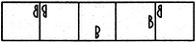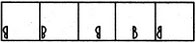In this case, the first five are the problem figures and you have to find the next one out of the five options given.

Find the next figure i.e. Analogy Type: In this category, you would be given 3 figures and you have to find the fourth figure, which has the same relation with the third figure as the second figure has with the first figure. Following is an example of this type:

Problem Figure: